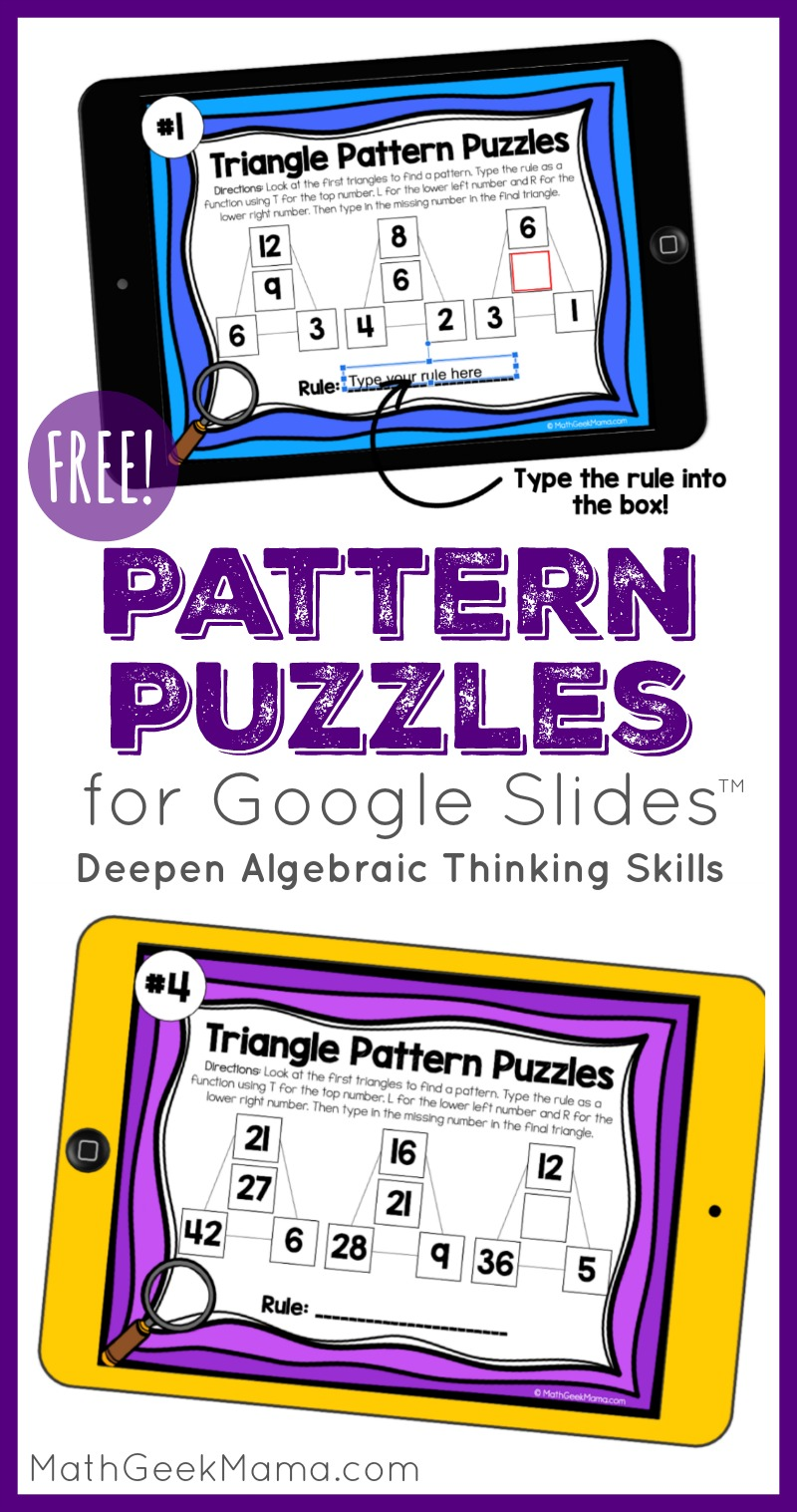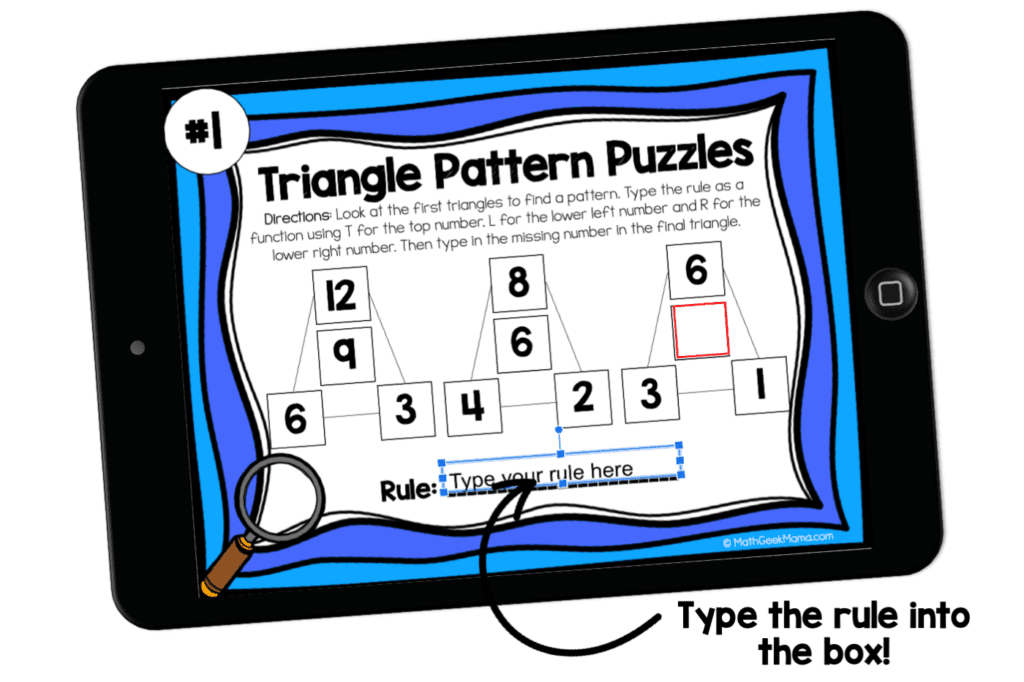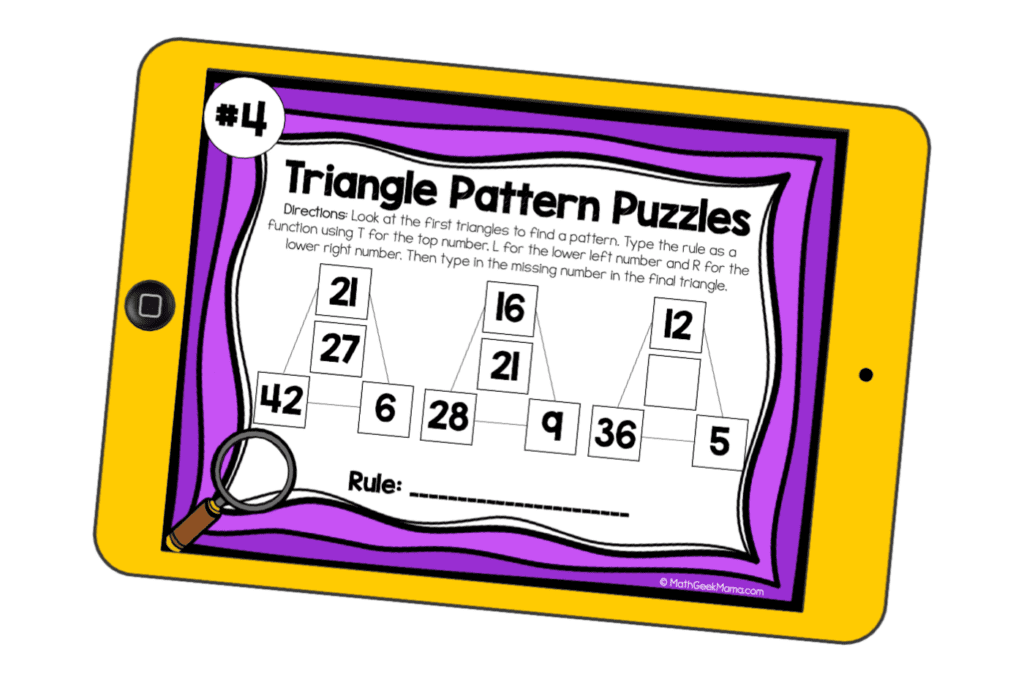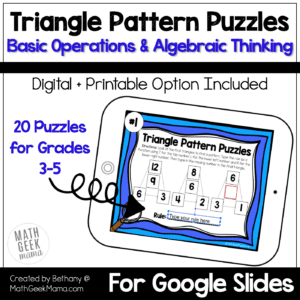# Pattern Puzzles to Build Algebraic Thinking | DIGITAL Puzzles

Do your kids see the connection between arithmetic and algebra? Build fluency with operations and problem solving skills with these engaging pattern puzzles for grades 3-5.

Mathematics is often referred to as the science of patterns. Noticing patterns in numbers, operations and the world around us is what math is all about. So I love finding unique ways to practice looking for patterns with my kids. These triangle pattern puzzles introduce the idea of a function or ‘rule’ in a fun and non-threatening way, while challenging kids to see patterns and practice basic operations. Once kids determine the ‘rule’ of the puzzle they can solve for the missing number. It’s such a fun and unique challenge, they won’t even realize they’re doing math!## How the Pattern Puzzles Work:

Each puzzle shows three triangles.

The numbers in the triangle (the top, left and right values) follow a particular rule to give the solution in the middle of the triangle.

The goal is for students to figure out what operations they can use to get the middle number.

For example, in puzzle #1, the numbers in the first triangle are 12, 6, & 3 and the solution in the middle is 9. Using addition & subtraction, we can use the triangle numbers to find the solution: 12 – 6 + 3 = 9.To test this pattern, we can follow the same rule with the middle triangle to see if the rule holds true. This gives us: 8 – 4 + 2 = 6 (this is true, so we have a pattern).

Following this pattern, we can now find the missing number in the last triangle: 6 – 3 + 1 = 4.

To write a general rule for this pattern, we can use the letters T (for the top number), L (for the bottom left number) and R (for the bottom right number).

This makes the rule for this pattern: T – L + R

An additional example, with directions is included in the Google Slides resource, helping to clarify the directions for students (especially if you are not completing these together in person).

## Completing the Pattern Puzzles in Google Slides:

This free download includes 5 different pattern puzzles in a digital format. By grabbing the Google Slides, you can assign one or more puzzles in Google Classroom or you can display it virtually with your class to discuss as a whole group.To complete them, there is space on each slide for students to type a general rule to represent the pattern they see and a box to type in the missing number.

Each of these 5 puzzles can be solved using only addition & subtraction.

An answer key is also included.

These would make fun math warm-ups or weekly puzzle challenges. You could also use these as enrichment for advanced students or early finishers.

Note: Although these are meant to be a no-print activity, where students type the rule and missing number onto each slide, you can print the slides out if you prefer.

To do this, make a copy of the resource in your Google Drive.

Then delete the text box on each slide where it says “Type your rule here.”

Then go to File–>Print and select your print settings.

## Pattern Puzzles for the Whole Year:

If you and your students enjoy these math challenges, you may be interested in the whole set of puzzles.

The complete set includes 20 different puzzles that increase in difficulty.These incorporate more math operations (multiplication & division), a “create you own puzzle” challenge and come in both digital + printer-friendly formats.

To try out this sample set of triangle pattern puzzles, use the link below to grab it from my shop!

And find more missing number puzzles at the links below:

### Never Run Out of Fun Math Ideas

If you enjoyed this post, you will love being a part of the Math Geek Mama community! Each week I send an email with fun and engaging math ideas, free resources and special offers. Join 163,000+ readers as we help every child succeed and thrive in math! PLUS, receive my FREE ebook, 5 Math Games You Can Play TODAY, as my gift to you!